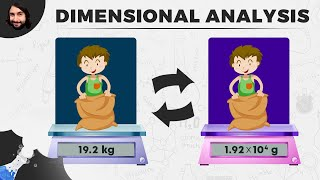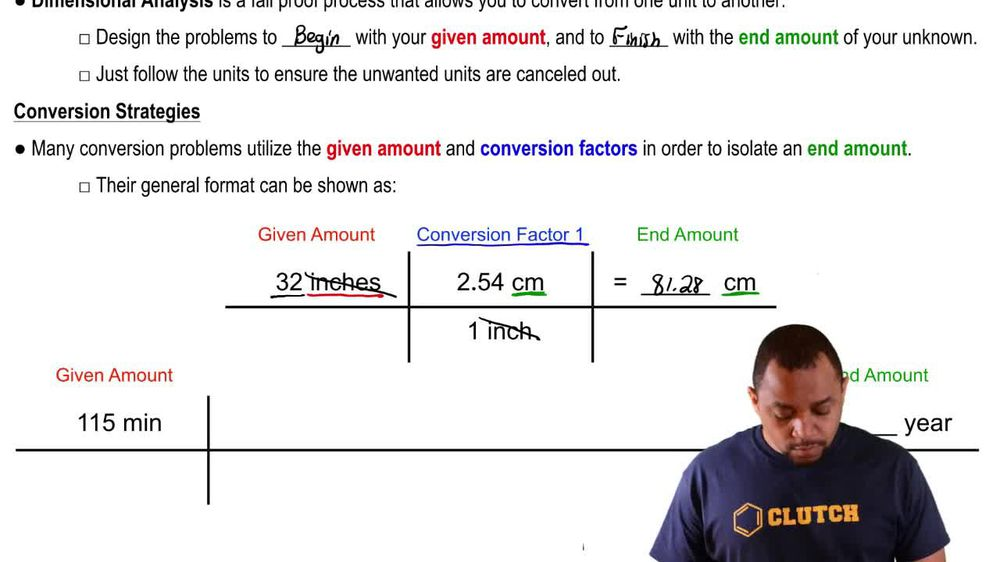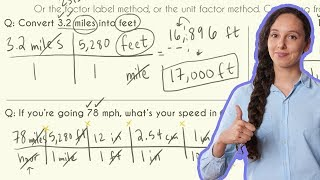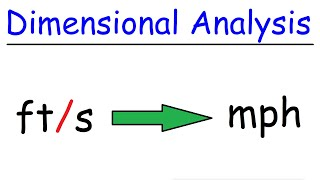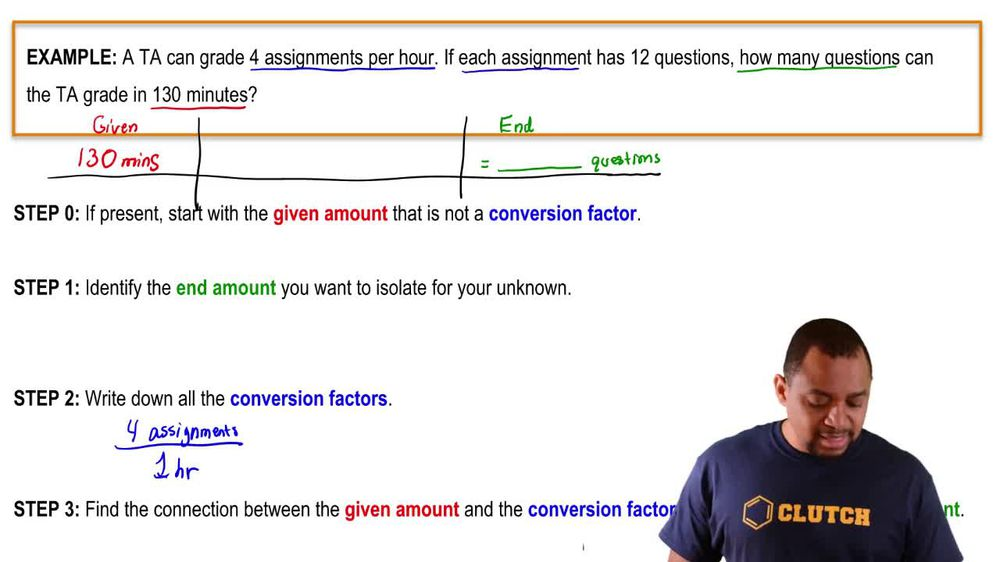Start typing, then use the up and down arrows to select an option from the list.
1. 1. Intro to General Chemistry2. Dimensional Analysis
Problem

# The density of polypropylene, a plastic commonly used to make bottle caps, yogurt containers, and carpeting, is 0.55 oz/in3. Calculate the density in units of g/cm3

Relevant Solution1m
Play a video:
Hello everyone today. We have the following problem polystyrene, a plastic used for packing peanuts, bottles and disposable utensils has a density of £61.18 per feet cubed. Convert this density two g per centimeters cubed. So the first thing you do is you want to write are given which is our 61.18 pounds per feet cubed. We know we have to get two g and to do that we can use the conversion factor that there are 454 g for every £1. And so our units for pounds would cancel out. We then want to use the conversion factor That will have one ft For every 12". And then we can convert the inches into cm by seeing that every one Is comprised of 2.54 cm. And since our answers and units of cubed, we're going ahead and put it, we're going to go ahead and put a three is the exponent there. And sorry, units 4" will cancel out in our units for feet cubed will cancel out. Maybe we'll be left with 0. grams per centimeter cubed as our final answer. I hope this helped. And until next time